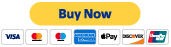Search

# Planck Units Ratios & the Electric Gravitational Force Ratio

Updated: Dec 26, 2019

In the exploration of Grand Unified Theories and/or Universal constants and ratios, one rather quickly gets exposed to the Planck constants. While contemplating unifying the current models, I began to think more and more that the Planck constants are the “most fundamental”, and I wondered how many other existing constants, concepts, and parameters within physics could be written in terms of those Planck constants. Once I realized how many other items indeed could be put into these terms, I then wondered why I never see it presented that way in texts, which in turn, led me to pursue it further.

Planck’s constants are determined by modeling energy as quantized and proportional to frequency, and by setting equal 2 quantities for minimal size and observation of black holes and energetic particle emissions. By that token, they seem like the foundation for unifying both relativity and quantum mechanics, yet are not normally presented as such by default. This post will not go into all the items that can be made from the Planck constants, I’m still finding more, but will rather focus on a few of the first, most prevalent relationships that were found, as well as use them to suggest a rather “Newtonian” ratio that can be explored for the unification of electromagnetism with gravity. The former other relations may eventually be developed and discussed at a later time.

To begin, start with the Planck Mass, (m_P) = 5.5 x 10^-8 kg, the Planck Length, (l_P) = 4 x 10^-35 m, and the Planck Time, (t_P) = 1.35 x 10^-43 s.

The first item that can be made directly from these is Planck’s general constant h. It turns out that h is equal to, (h) ≡ m_P * (l_P)^2 / t_P. This is interesting in that h is usually given as a standalone physical constant unto itself, when in fact it turns out that it can be viewed as dependent on 3 more fundamentally independent constants.

Second, is what turned out to me to be the most interesting and simple construction of a constant. Did you know that the speed of light is actually just a construction of the Planck constants? Simply divide the Planck length by the Planck time, and voila, you have the speed of light, (c) ≡ l_P / t_P.

The next relationship comes in a pair as a product of 2 constants. It turns out that the product of the Permittivity of free space (ε0) and the Permeability of free space (μ0) is simply the ratio of the squares of the Planck time and length. That is, that ε0 * μ0 ≡ (t_P)^2 / (l_P)^2.

The last 2 constants for this post that are discussed in terms of Planck constants are the Gravitational force constant and Coulomb’s Electric force constant. The Gravitational force constant (G) works out to be, (G) ≡ (l_P)^3 / m_P*(t_P)^2, and the Coulomb’s Electric force constant K_e is, (K_e) ≡ (l_P)^3 * μ0 / 4π(t_P)^2. In that form, the electric force constant introduces π and μ0 into the mix.

So what can be done from there with these constructions? For fun, I decided to try and unify electromagnetism with gravity in the most basic, Newtonian, that is just to say algebraic, way possible. Noting that the 2 basic formulae for the Gravitational and Electric Forces are both identical inverse square laws, with the only difference being the constants and charge versus mass, I attempted to describe an electric force to gravitational force ratio. That is, given F_C = K_e * q1q2 / r2, and F_G = G * m1m2 / r2, let the gravitational electromagnetic force ratio be F_GEM ≡ F_C / F_G = μ0 m_P q1q2 / 4π l_P m1m2 = 1.3467 x 10^20 * q1q2 / m1m2.

At that point, I’m not sure how useful that specific construction becomes, as it suggests a constant ratio between 2 particles of different charges and masses, which has no general basis of charge or mass being dictated by the other. However, it’s fun to think about, and could point to specific cases where it is such or where that ratio value could be useful.

That’s it for now. I may take this topic further in the future, but for now, if you would like to add to this idea, and or discover something novel while you do, I would be interested to know. Also, if you are interested in further reading about the topic in general, I suggest starting with the Wiki page on Natural Units, found here.

9 views

### Recent Posts

See All

#### Information Theory

1.Information Theories a)This is one model for the measuring the number of facts of information surrounding a human over a given time, K(t). It does this in a numerical fashion and considers neither

#### Cosmic Commentary

Cosmic Commentary This is a platform for refining coinciding physics, linguistic, and mathematical symbolisms, and applying such towards the pursuit of single fundamental “particle” theories. Contents© 2017- 2020 by Thoughtfarm. Proudly created with Wix.com